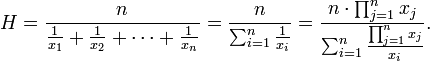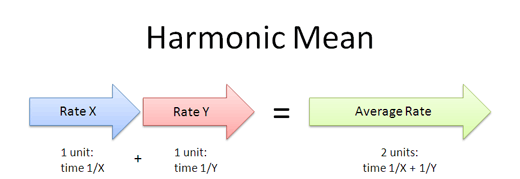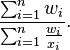×#### Thank you for registering.

One of our academic counsellors will contact you within 1 working day.

Click to Chat

1800-1023-196

+91-120-4616500

CART 0

• 0

MY CART (5)

Use Coupon: CART20 and get 20% off on all online Study Material

ITEM
DETAILS
MRP
DISCOUNT
FINAL PRICE
Total Price: Rs.

There are no items in this cart.
Continue Shopping```Harmonic Mean

Table of Content

Formula for Harmonic Mean

Weighted Harmonic Mean

Some Important Remarks

Related ResourcesHarmonic Mean is one of the several kinds of average. Mathematically, the harmonic mean between two numbers a and b is defined as

H = 2/ (1/a + 1/b)

This can further be written as:

H = 2ab/(a+b)

Likewise, the harmonic mean of n positive numbers x1, x2, …. , xn is defined to beThe harmonic mean of two numbers is in fact the reciprocal of arithmetic mean of the reciprocal of the numbers. This simply means that if H is the harmonic mean between two numbers say a and b then 1/a, 1/H and 1/b are in A.P.

Let us consider a simple example to understand the concept of harmonic mean:

We wish to find the harmonic mean of 1, 2 and 4. Since these are three in number, so by applying the formula the H.M. is given by

3/ (1/1 + 1/2 + 1/4) = 12/7.

Formula for Harmonic Mean

Let us see how exactly we reach the formula for harmonic mean between two numbers a and b:

Let H be the harmonic mean between two numbers a and b.

So, a, H, b are in H.P.

This means that 1/a, 1/H, 1/b are in A.P.

or, 1/H – 1/a = 1/b – 1/H.

or, 2/H = 1/a + 1/b

= a+b/ab

∴     H = 2ab/a+b

On the same lines, we may also find two harmonic means between two numbers.

Let us assume that Ha and Hb are two harmonic means between a and b.

Then it follows that a, Ha, Hb and b are in H.P.

Then 1/a, 1/Ha, 1/Hb, 1/b are in A.P.

Hence, using the formula we have

tn = a + (n-1)d

So, 1/b – 1/a = 3d, where‘d’ denotes the common difference of the A.P.

This implies that 3d = (a-b)/b

Hence, d = (a-b)/3ab

So, 1/H1 = 1/a + d = 1/a + a–b/3ab = a+2b/3ab

and 1/H2 = 1/a + 2d = 1/a + 2(a–b)/3ab = 2a+2b/3ab

Hence, these are the two harmonic means between a and b.Weighted Harmonic Mean

Another concept closely related to harmonic mean is that of weighted harmonic mean. If we have a set of weights w1, w2, …. , wn associated with the set of values x1, x2, …. , xn, then the weighted harmonic mean is defined asHarmonic mean is in fact a special case of weighted harmonic mean where all the weights are equal to 1 and is equal to any weighted harmonic mean having all equal weights.

The following figure shows two crossed ladders A and B with each having feet at the base of one side wall and one leaning against a wall at height A and the other leaning against the opposite wall at height B. in such a case, h is half the harmonic mean of A and B.Let us find the harmonic mean H of 2, 20, 10, 5, 1 using the formula stated above.

We explain the whole procedure in the form of steps:

1. First calculate the total number of items which in this case is 5 and hence, N = 5.

2. Now use the formula of harmonic mean i.e.

H = N / (1/a1 + 1/a2 + ….. 1/aN)

= 5/ (1/2 + 1/20 + 1/10 + 1/5 + 1/1)

= 5/ (37/20)

= 5 .20 / 37

= 100/37.

Watch this Video for more reference​

Some Important Remarks

• If a and b are two non-zero numbers, then the harmonic mean of a and b is a number H such that the numbers a, H, b are in H.P. We have H = 1/H = 1/2 (1/a + 1/b) ⇒ H = 2ab/a+b.

• If a1, a2, ……, an are n non-zero numbers. then the harmonic mean H of these number is given by 1/H = 1/n (1/a1 + 1/a2 +...+ 1/an).

• The n numbers H1, H2, ……, Hn are said to be harmonic means between a and b, if a, H1, H2 ……, Hn, b are in H.P. i.e. if 1/a, 1/H1, 1/H2, ..., 1/Hn, 1/b are in A.P. Let d be the common difference of the A.P., Then 1/b = 1/a + (n+1) d ⇒ d = a–b/(n+1)ab.

Thus 1/H1 = 1/a + a–b/(n+1)ab,

1/H2 = 1/a + 2(a–n)/(n+1)ab,

………..     ……….

1/Hn = 1/a + n(a–b)/(n+1)ab.

askIITians offers comprehensive study material which covers all the important topics of IIT JEE Mathematics. The concepts of mean including arithmetic mean, geometric mean and harmonic mean have been covered in detail along with various numerical. It is important to have a strong hold on the topic in order to remain competitive in the JEE.

Related Resources

Look into the Previous Year Papers with Solutions to get a hint of the kinds of questions asked in the exam.

You can get the knowledge of Important Books of Mathematics here.

You may also like to refer Arithmetic Mean and Geometric Mean.

To read more, Buy study materials of Sequences and Series comprising study notes, revision notes, video lectures, previous year solved questions etc. Also browse for more study materials on Mathematics here.
```### Course Features

• 731 Video Lectures
• Revision Notes
• Previous Year Papers
• Mind Map
• Study Planner
• NCERT Solutions
• Discussion Forum
• Test paper with Video Solution